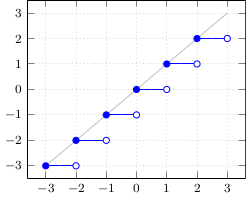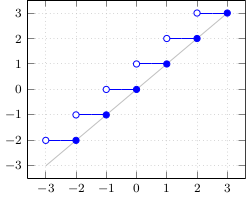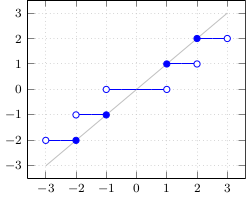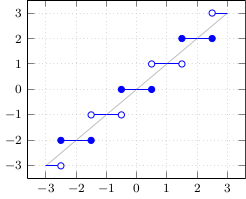## C/C++ rounding cheat sheet

### Rounding-mode independent methods

#### Floor• double floor(double x);
• float floorf(float x);
• (long int)floor(x); / (long int)floorf(x);
• Corresponding rounding mode: FE_DOWNWARD

#### Ceil• double ceil(double x);
• float ceilf(float x);
• (long int)ceil(x); / (long int)ceilf(x);
• Corresponding rounding mode: FE_UPWARD

#### Truncate / Round toward zero• double trunc(double x);
• float truncf(float x);
• (long int)x;
• Corresponding rounding mode: FE_TOWARDZERO

#### Round to nearest, halfway cases away from zero• double round(double x);
• float roundf(float x);
• long int lround(double x);
• long int lroundf(float x);
• (long int)(x+0.5) - has problems with <0 and (e.g. float) >=223, etc. !

#### Round to nearest, halfway cases to even• (rint / nearbyint / lrint with default rounding mode)
• Corresponding rounding mode: FE_TONEAREST (default)
Less commonly available:
• double roundeven(double x);
• float roundevenf(float x);
• (long int)roundeven(x); / (long int)roundevenf(x);

#### Round to nearest, halfway cases upwards

• (long int)floor(x+0.5); / (long int)floorf(x+0.5f); - TODO: better...?
• ... you probably should use halfway-to-even!

### Rounding-mode dependent methods

• rint* - does not raise FE_INEXACT
• nearbyint* - raises FE_INEXACT
• lrint* - FE_INEXACT is also a domain error (FE_INVALID)

### Other languages

#### Javascript

• x|0;   => trunc/cast (limited to 32 bit!)
• Math.round(x);   => Round to nearest, halfway cases upwards
• x.fixed(digits);   - halfway cases are not fully reliable!
• Math.floor(x); / Math.ceil(x);

#### PHP

• \$x|0; / intval(\$x) / (int)\$x / (integer)\$x;   => trunc/cast (limited to 32 / 64 bit!)
• round(\$x,\$precision=0,\$mode=PHP_ROUND_HALF_UP)   - \$mode=_HALF_UP | _HALF_DOWN | _HALF_EVEN | _HALF_ODD since PHP 5.3
• floor(\$x); / ceil(\$x);